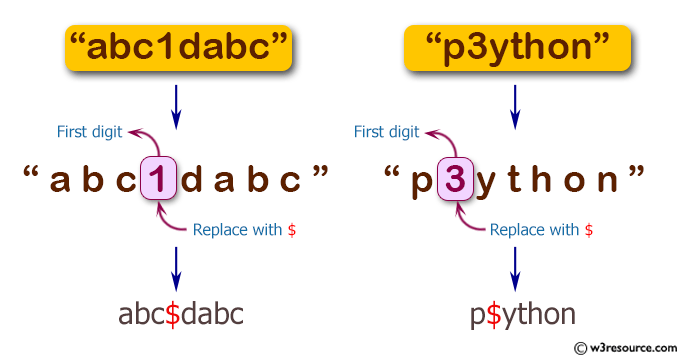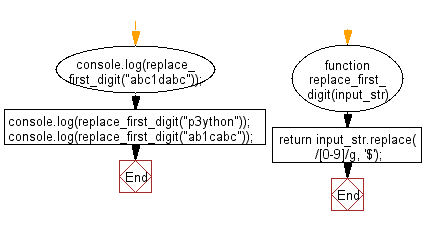# JavaScript: Replace the first digit in a string (should contains at least digit) with \$ character

## JavaScript Basic: Exercise-136 with Solution

Write a JavaScript program to replace the first digit in a string (should contains at least digit) with \$ character.

Pictorial Presentation:Sample Solution:

HTML Code:

``````<!DOCTYPE html>
<html>
<meta charset="utf-8">
<meta name="viewport" content="width=device-width">
<title>Replace the first digit in a string (should contains at least digit) with \$ character</title>
<body>

</body>
</html>
```
```

JavaScript Code:

``````function replace_first_digit(input_str) {

return input_str.replace(/[0-9]/, '\$');
}
console.log(replace_first_digit("abc1dabc"));
console.log(replace_first_digit("p3ython"));
console.log(replace_first_digit("ab1cabc"));
``````

Sample Output:

```abc\$dabc
p\$ython
ab\$cabc
```

Flowchart:ES6 Version:

``````function replace_first_digit(input_str) {

return input_str.replace(/[0-9]/, '\$');
}
console.log(replace_first_digit("abc1dabc"));
console.log(replace_first_digit("p3ython"));
console.log(replace_first_digit("ab1cabc"));
``````

Live Demo:

See the Pen javascript-basic-exercise-136 by w3resource (@w3resource) on CodePen.

Improve this sample solution and post your code through Disqus

What is the difficulty level of this exercise?

Test your Programming skills with w3resource's quiz.

﻿

## JavaScript: Tips of the Day

Checks if a string is an anagram of another string (case-insensitive, ignores spaces, punctuation and special characters)

Example:

```const isAnagram = (str1, str2) => {
const normalize = str =>
str
.toLowerCase()
.replace(/[^a-z0-9]/gi, '')
.split('')
.sort()
.join('');
return normalize(str1) === normalize(str2);
};
console.log(isAnagram('iceman', 'cinema')); // true
```

Output:

```true
```